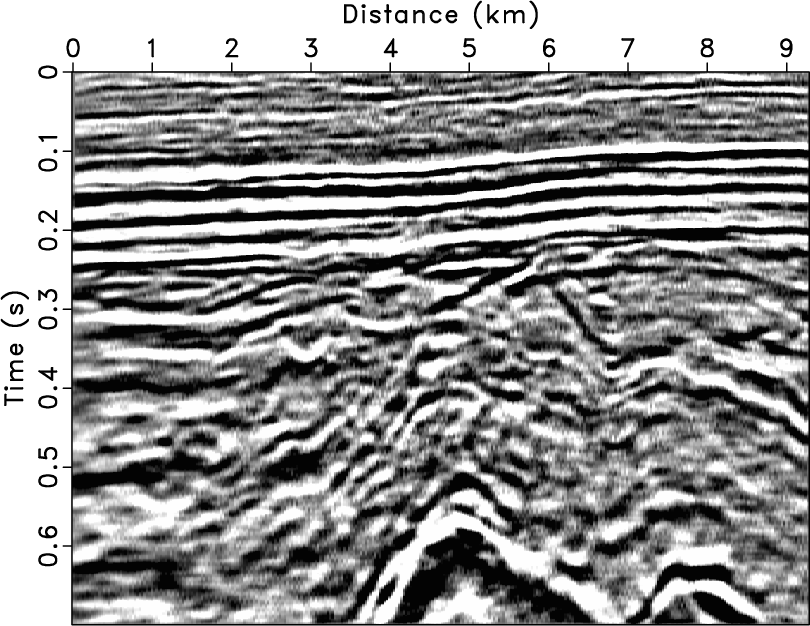In seismic exploration there are many sources of random noise, for example, scattering from a complex surface. Prediction filters (PFs) have been widely used for random noise attenuation, but these typically assume that the seismic signal is stationary. Seismic signals are fundamentally nonstationary. Stationary PFs fail in the presence of nonstationary events, even if the data are cut into overlapping windows (“patching”). We propose an adaptive PF method based on streaming and orthogonalization for random noise attenuation in the $t$-$x$ domain. Instead of using patching or regularization, the streaming orthogonal prediction filter (SOPF) takes full advantage of the streaming method, which generates the signal value as each new noisy data value arrives. The streaming signal-and-noise orthogonalization further improves the signal recovery ability of the SOPF. The streaming characteristic makes the proposed method faster than iterative approaches. In comparison with $f$-$x$ deconvolution and $f$-$x$ regularized nonstationary autoregression (RNA), we tested the feasibility of the proposed method in attenuating random noise on two synthetic datasets. Field data examples confirmed that the $t$-$x$ SOPF had a reasonable denoising ability in practice.